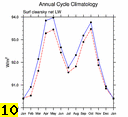SILHS_MG10_1800ts_10sc_QNZMAF_UWM_test
and
OBS data (info)

DIAG Set 10 - Annual cycle line plots of global means

ICEFRAC Sea-ice area (Northern) plot
ICEFRAC Sea-ice area (Southern) plot
SST Sea surface temperature plot

SMMR & SSM/I 1979-1999
ICEFRAC Sea-ice area (Northern) plot
ICEFRAC Sea-ice area (Southern) plot

Legates & Willmott 1920-80
PRECT Precipitation rate plot
TREFHT 2-meter air temperature plot

Willmott & Matsuura 1950-99
TREFHT 2-meter air temperature (land) plot

MERRA Analysis 1979-2013 (NASA)
PREH2O Total precipitable water plot
PSL Sea-level pressure plot
T 850 850 mb temperature plot
T 200 200 mb temperature plot
TS Surface temperature plot
U 200 200 mb zonal wind plot
Z 500 500 mb geopotential height plot
Z 300 300 mb geopotential height plot

ERA40 Reanalysis 1980-2001
EP Evaporation - precipitation plot
LHFLX Surface latent heat flux plot
PREH2O Precipitable water plot
QFLX Surface water flux plot
T 850 850 mb temperature plot
T 200 200 mb temperature plot
U 200 200 mb zonal wind plot
Z 500 500 mb geopotential height plot
Z 300 300 mb geopotential height plot

ERA-Interim Reanalysis 1989-2005
PREH2O Precipitable water plot
T 850 850 mb temperature plot
T 200 200 mb temperature plot
U 200 200 mb zonal wind plot
Z 500 500 mb geopotential height plot
Z 300 300 mb geopotential height plot

JRA25 Reanalysis 1979-04
PREH2O Total precipitable water plot
PSL Sea-level pressure plot
SHFLX Surface sensible heat flux plot
T 850 850 mb temperature plot
T 200 200 mb temperature plot
TREFHT 2-meter surface air temperature plot
U 200 200 mb zonal wind plot
Z 500 500 mb geopotential height plot
Z 300 300 mb geopotential height plot

AIRS IR Sounder 2002-06
T 850 * 850 mb temperature plot
T 200 200 mb temperature plot

Large-Yeager 1984-2004
SHFLX Surface sensible heat flux plot
QFLX Surface water flux plot
FLNS Surf Net LW flux plot
FSNS Surf Net SW flux plot

NVAP 1988-1999
PREH2O Total precipitable water plot
TGCLDLWP Cloud liquid water (ocean) plot

Woods Hole OAFLUX 1958-2006
LHFLX Latent Heat Flux plot
QFLX Surface Water Flux plot

GPCP 1979-2009
PRECT Precipitation rate plot

CMAP (Xie-Arkin) 1979-98
PRECT Precipitation rate plot

UWisc (v3) 1988-2008
TGCLDLWP cloud liquid water (ocean) plot

SSM/I (Wentz) 1987-2000
PRECT Precipitation rate (ocean) plot
PREH2O Precipitable water (ocean) plot

TRMM (3B43) 1998-2009
PRECT Precipitation rate (38S-38N) plot

ERBE Feb1985-Apr1989
FLUT TOA upward longwave flux plot
FLUTC TOA clearsky upward longwave flux plot
FSNTOA TOA net shortwave flux plot
FSNTOAC TOA clearsky net shortwave flux plot
LWCF TOA longwave cloud forcing plot
RESTOA TOA residual energy flux plot
SWCF TOA shortwave cloud forcing plot

CERES-EBAF March 2000-Feb 2013
ALBEDO TOA albedo plot
ALBEDOC TOA clearsky albedo plot
FLUT * TOA upward LW flux plot
FLUTC TOA clearsky upward LW flux plot
FSNTOA * TOA net SW flux plot
FSNTOAC TOA clearsky net SW flux plot
LWCF * TOA longwave cloud forcing plot
SWCF * TOA shortwave cloud forcing plot

CERES 2000-2003
ALBEDO TOA albedo plot
ALBEDOC TOA clearsky albedo plot
FLUT * TOA upward LW flux plot
FLUTC TOA clearsky upward LW flux plot
FSNTOA * TOA net SW flux plot
FSNTOAC TOA clearsky net SW flux plot
LWCF * TOA longwave cloud forcing plot
SWCF * TOA shortwave cloud forcing plot

ISCCP FD Jul1983-Dec2000
FLDS Surface LW downwelling flux plot
FLNS Surface Net LW flux plot
FSDS Surface SW downwelling flux plot
FSNS Surface Net SW flux plot

ISCCP D2 1983-2001
CLDHGH (IR clouds) High-level cloud amount plot
CLDHGH (VIS/IR/NIR clouds) High-level cloud amount plot
CLDLOW (IR clouds) Low-level cloud amount plot
CLDLOW (VIS/IR/NIR clouds) Low-level cloud amount plot
CLDMED (IR clouds) Medium-level cloud amount plot
CLDMED (VIS/IR/NIR clouds) Medium-level cloud amount plot
CLDTOT (IR clouds) Total cloud amount plot
CLDTOT (VIS/IR/NIR clouds) Total cloud amount plot

CFMIP Observation Simulator Package (COSP) Primary Diagnostics

ISCCP-COSP From D1 Daytime Jul1983-Jun2008

CALIPSO GOCCP 2007-2010
CLDTOT_CAL Total cloud amount plot
CLDLOW_CAL Low-level cloud amount plot
CLDMED_CAL Mid-level cloud amount plot
CLDHGH_CAL High-level cloud amount plot

ISCCP-COSP From D1 Daytime Jul1983-Jun2008
CLDTOT_ISCCPCOSP Total cloud amount (tau > 0.3) plot
CLDTHICK_ISCCPCOSP Optically thick cloud amount (tau > 23.0) plot

MISR L3 CTH-OD V5 Mar2000-Nov2009
CLDTOT_MISR Total cloud amount (tau > 0.3) plot
CLDTHICK_MISR Optically thick cloud amount (tau > 23.0) plot

MODIS-COSP 2003-2010
CLDTOT_MODIS Total cloud amount (tau > 0.3) plot
CLDTHICK_MODIS Optically thick cloud amount (tau > 23.0) plot

CALIPSO GOCCP 2007-2010
CLDTOT_CAL Total cloud amount plot
CLDLOW_CAL Low-level cloud amount plot
CLDMED_CAL Mid-level cloud amount plot
CLDHGH_CAL High-level cloud amount plot

CLOUDSAT-COSP From Level 2 GEOPROF Jun2006-Nov2010
CLDTOT_CS2 Total cloud amount plot

ISCCP-COSP From D1 Daytime Jul1983-Jun2008
CLDTOT_ISCCPCOSP Total cloud amount (tau > 0.3) plot
CLDLOW_ISCCPCOSP Low-topped cloud amount (tau > 0.3) plot
CLDMED_ISCCPCOSP Mid-topped cloud amount (tau > 0.3) plot
CLDHGH_ISCCPCOSP High-topped cloud amount (tau > 0.3) plot
CLDTHICK_ISCCPCOSP Optically thick cloud amount (tau > 23.0) plot
MEANPTOP_ISCCPCOSP Mean cloud top pressure plot
MEANCLDALB_ISCCPCOSP Mean cloud albedo plot

MISR L3 CTH-OD V5 Mar2000-Nov2009
CLDTOT_MISR Total cloud amount (tau > 0.3) plot
CLDLOW_MISR Low-topped cloud amount (tau > 0.3) plot
CLDMED_MISR Mid-topped cloud amount (tau > 0.3) plot
CLDHGH_MISR High-topped cloud amount (tau > 0.3) plot
CLDTHICK_MISR Optically thick cloud amount (tau > 23.0) plot

MODIS-COSP 2003-2010
CLDTOT_MODIS Total cloud amount (tau > 0.3) plot
CLDLOW_MODIS Low-topped cloud amount (tau > 0.3) plot
CLDMED_MODIS Mid-topped cloud amount (tau > 0.3) plot
CLDHGH_MODIS High-topped cloud amount (tau > 0.3) plot
CLDTHICK_MODIS Optically thick cloud amount (tau > 23.0) plot
CLIMODIS Total ice cloud amount plot
CLWMODIS Total liquid cloud amount plot
IWPMODIS Mean ice water path plot
LWPMODIS Mean liquid water path plot
PCTMODIS Mean cloud top pressure plot
REFFCLIMODIS Mean effective radius (ice clouds) plot
REFFCLWMODIS Mean effective radius (liquid clouds) plot
TAUILOGMODIS Log-weighted mean optical thickness (ice clouds) plot
TAUWLOGMODIS Log-weighted mean optical thickness (liquid clouds) plot
TAUTLOGMODIS Log-weighted mean optical thickness (all clouds) plot
TAUIMODIS Mean optical thickness (ice clouds) plot
TAUWMODIS Mean optical thickness (liquid clouds) plot
TAUTMODIS Mean optical thickness (all clouds) plot